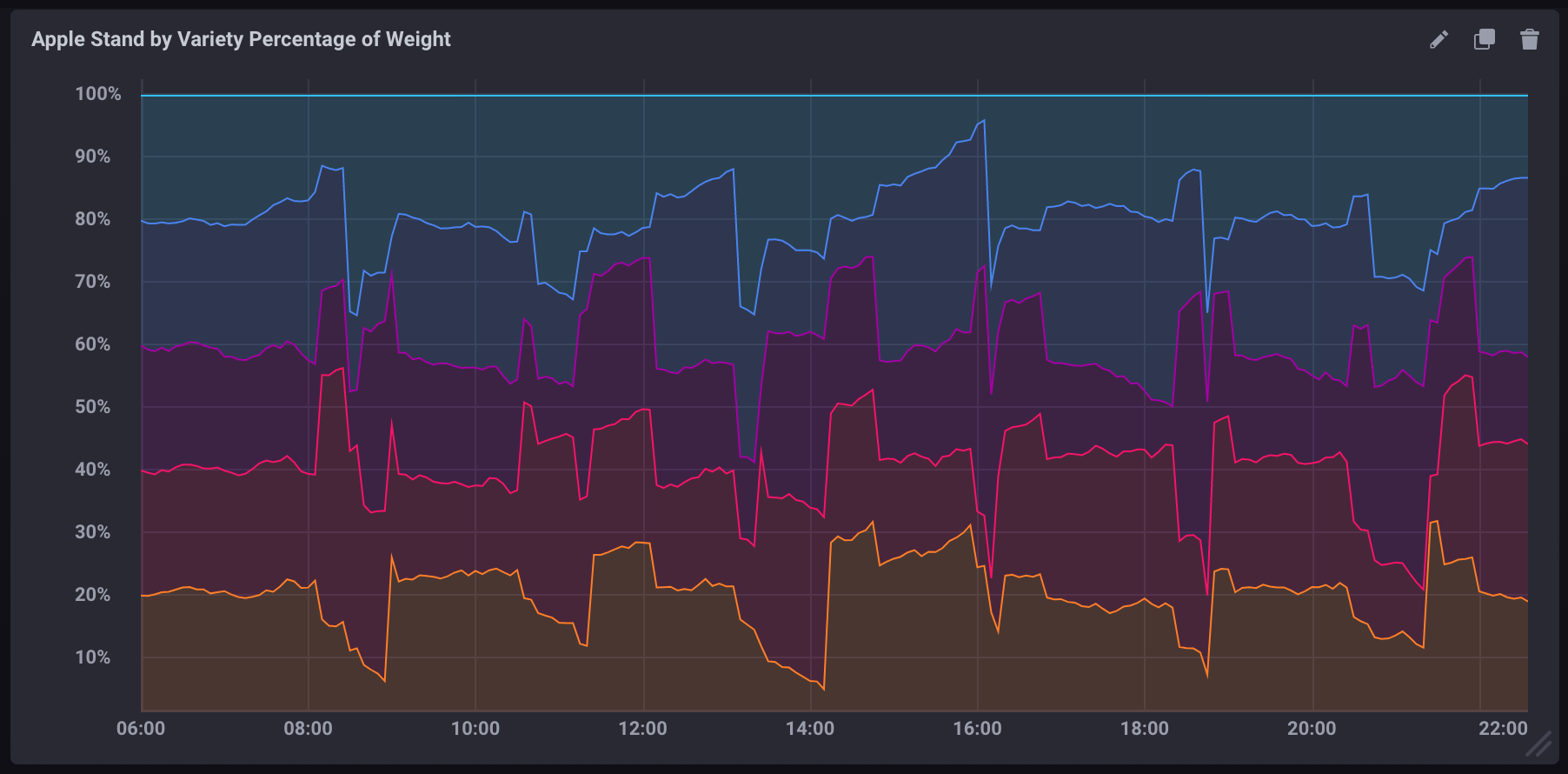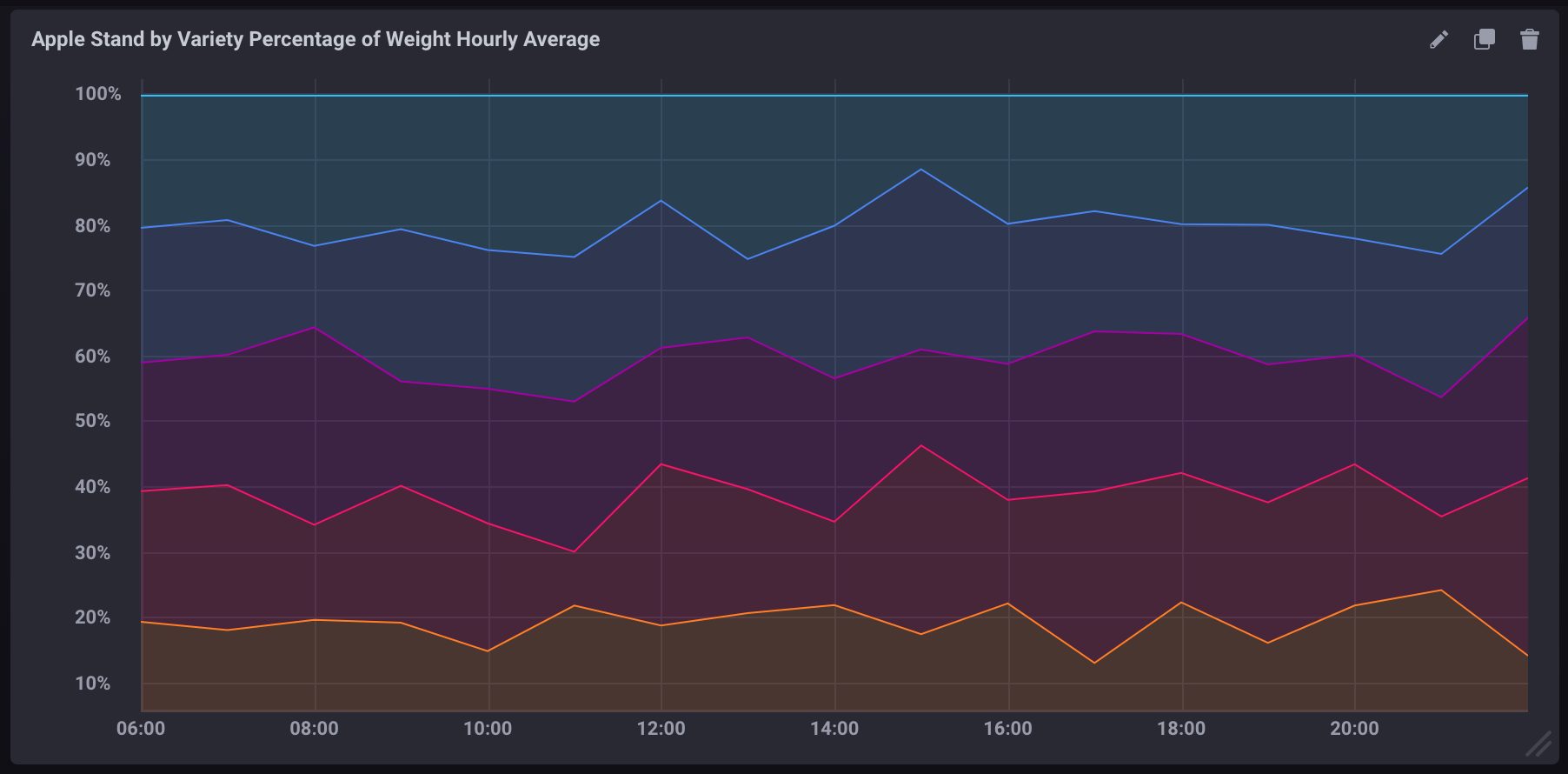# Calculating percentages in a query

InfluxQL lets you perform simple math equations which makes calculating percentages using two fields in a measurement pretty simple. However there are some caveats of which you need to be aware.

## Basic calculations within a query

`SELECT` statements support the use of basic math operators such as `+`,`-`,`/`, `*`, `()`, etc.

``````-- Add two field keys
SELECT field_key1 + field_key2 AS "field_key_sum" FROM "measurement_name" WHERE time < now() - 15m

-- Subtract one field from another
SELECT field_key1 - field_key2 AS "field_key_difference" FROM "measurement_name" WHERE time < now() - 15m

-- Grouping and chaining mathematical calculations
SELECT (field_key1 + field_key2) - (field_key3 + field_key4) AS "some_calculation" FROM "measurement_name" WHERE time < now() - 15m``````

## Calculating a percentage in a query

Using basic math functions, you can calculate a percentage by dividing one field value by another and multiplying the result by 100:

``SELECT (field_key1 / field_key2) * 100 AS "calculated_percentage" FROM "measurement_name" WHERE time < now() - 15m``

## Calculating a percentage using aggregate functions

If using aggregate functions in your percentage calculation, all data must be referenced using aggregate functions. You can’t mix aggregate and non-aggregate data.

All Aggregate functions need a `GROUP BY time()` clause defining the time intervals in which data points are grouped and aggregated.

``SELECT (sum(field_key1) / sum(field_key2)) * 100 AS "calculated_percentage" FROM "measurement_name" WHERE time < now() - 15m GROUP BY time(1m)``

## Examples

#### Sample data

The following example uses simulated Apple Stand data that tracks the weight of baskets containing different varieties of apples throughout a day of business.

2. Import the sample data:

``influx -import -path=path/to/apple_stand.txt -precision=s -database=apple_stand``

### Calculating percentage of total weight per apple variety

The following query calculates the percentage of the total weight each variety accounts for at each given point in time.

``````SELECT
("braeburn"/total_weight)*100,
("granny_smith"/total_weight)*100,
("golden_delicious"/total_weight)*100,
("fuji"/total_weight)*100,
("gala"/total_weight)*100
FROM "apple_stand"."autogen"."variety"``````
\*

If visualized as a stacked graph in Chronograf, it would look like:### Calculating aggregate percentage per variety

The following query calculates the average percentage of the total weight each variety accounts for per hour.

``````SELECT
(mean("braeburn")/mean(total_weight))*100,
(mean("granny_smith")/mean(total_weight))*100,
(mean("golden_delicious")/mean(total_weight))*100,
(mean("fuji")/mean(total_weight))*100,
(mean("gala")/mean(total_weight))*100
FROM "apple_stand"."autogen"."variety"
WHERE time >= '2018-06-18T12:00:00Z' AND time <= '2018-06-19T04:35:00Z'
GROUP BY time(1h)``````

• It uses aggregate functions (`mean()`) for pulling all data.
• It includes a `GROUP BY time()` clause which aggregates data into 1 hour blocks.
• It includes an explicitly limited time window. Without it, aggregate functions are very resource-intensive.

If visualized as a stacked graph in Chronograf, it would look like:New! Cloud or OSS?

### InfluxDB OSS 2.0 release candidate

InfluxDB OSS v2.0.rc includes breaking changes that require a manual upgrade from all alpha and beta versions. For information, see: